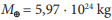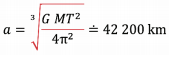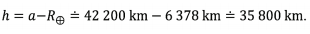# Practical exercise 8: GEOSTATIONARY SATELLITE

A geostationary satellite is a satellite that Earthlings see "hanging" motionless in the sky. The satellite must be in the plane of the equator and orbit along the circle in the same direction as the direction of rotation of the Earth about its axis, see Fig. 7 and 8.

a) Explain why it is not possible for the satellite to move in another plane.

b) The mass of the Earth isand rotation around the axis takes T = 24 hod. Determine how high the satellite orbits above the Earth's surface.Fig. 7 https://www.esa.int/spaceinimages/Images/2009/03/Geostationary_orbit, cit. 05.07.2018Fig. 8 From the observer's point of view, the geostationary satellite "“hangs" over one point on the Earth's surface (illustrative image)

Solution:

a) The plane of a satellite´s orbit must pass through the centre of the Earth (mass centre). If the satellite, from the point of view of an observer connected to the Earth's surface, is to always be at the same point, it must rotate in the direction of the Earth's rotation. However, this means that the plane of rotation must be perpendicular to the axis of rotation. It is then clear from the geometric idea that there is only one such plane.

b) The semi major axis (radius of a circular trajectory) is found by 3rd Kepler's lawThe centre of the circular orbit of the satellite lies in the centre of the Earth (in the mass centre or focus).

The distance of the satellite above the Earth's surface is then: